# GSEB Solutions Class 12 Maths Chapter 7 Integrals Ex 7.1

Gujarat Board GSEB Textbook Solutions Class 12 Maths Chapter 7 Integrals Ex 7.1 Textbook Questions and Answers.

## Gujarat Board Textbook Solutions Class 11 Maths Chapter 7 Integrals Ex 7.1

Find an antiderivative (or integral) of the following by the method of inspection:
Question 1.
sin 2x
Solution:
We know that $$\frac{d}{dx}$$ cos 2x = – 2sin 2x.
or $$\frac{d}{dx}$$ (- $$\frac{1}{2}$$ cos 2x) = sin 2x
∴ ∫sin 2x dx = – $$\frac{1}{2}$$ cos 2x + C.

Question 2.
cos 3x
Solution:
2. We know that $$\frac{d}{dx}$$ (sin 3x) = 3 cos 3x.
⇒ cos 3x = $$\frac{1}{3}$$ $$\frac{d}{dx}$$ (sin 3x)
⇒ cos 3x = $$\frac{d}{dx}$$ ($$\frac{1}{3}$$ sin 3x)
∴ An antiderivative of cos 3x is $$\frac{1}{3}$$ sin 3x + C.

By using free Taylor Series Calculator, you can easily find the approximate value of the integration function

Question 3.
e2x
Solution:
We know that
$$\frac{d}{dx}$$(e2x) = 2e2x.
⇒ e2x = $$\frac{1}{2}$$ $$\frac{d}{dx}$$ (e2x)
⇒ e2x = $$\frac{d}{dx}$$ ($$\frac{1}{2}$$ e2x)
∴ An antiderivative of e2x is $$\frac{1}{2}$$ e2x + C.

Question 4.
(ax + b)
2
Solution:
We know that $$\frac{d}{dx}$$ (ax + b)3 = 3a(ax + b)2.
⇒ (ax + b)2 = $$\frac{1}{3a}$$ $$\frac{d}{dx}$$(ax + b)3
⇒ (ax + b)2 = $$\frac{d}{dx}$$[$$\frac{1}{3a}$$(ax + b)3]
∴ An antiderivative of (ax + b)2 = $$\frac{1}{3a}$$(ax + b)3 + C.

Question 5.
sin 2x – 4e
3x
Solution:
We know that $$\frac{d}{dx}$$(cos 2x) = – 2 sin 2x.
⇒ sin 2x = $$\frac{d}{dx}$$(-$$\frac{1}{2}$$ cos 2x)
and $$\frac{d}{dx}$$(4e3x) = 4 × 3e3x
⇒ 4e3x = $$\frac{d}{dx}$$($$\frac{1}{3}$$ e3x)
∴ An antiderivative of sin 2x – 4e3x is – $$\frac{1}{2}$$ cos 2x – $$\frac{4}{3}$$e3x + C.Find the following integrals:
Question
6. ∫(4e3x + 1)dx
Solution: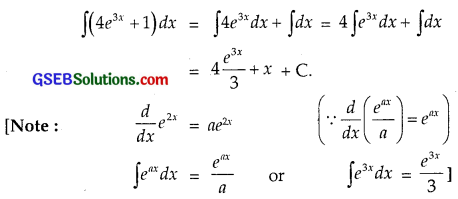Question 7.
∫x2(1 – $$\frac{1}{x^{2}}$$)dx
Solution: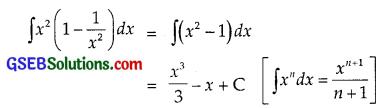Question 8.
∫(ax2 + bx + c)dx
Solution: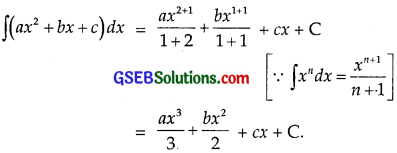Question 9.
∫(2x2 + ex) dx
Solution: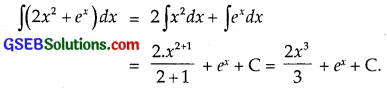Question 10.
∫($$\sqrt{x}$$ – $$\frac{1}{\sqrt{x}}$$)2 dx
Solution: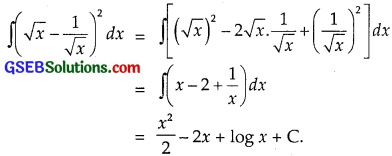Question 11.
∫$$\frac{x^{3}+5 x^{2}-4}{x^{2}}$$dx
Solution: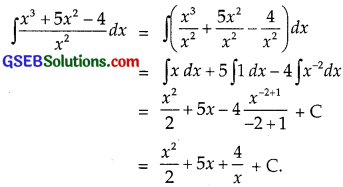Question 12.
∫$$\frac{x^{3}+3 x+4}{\sqrt{x}}$$dx
Solution: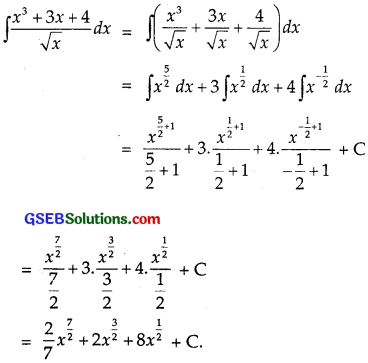Question 13.
∫$$\frac{x^{3}-x^{2}+x-1}{x-1}$$dx
Solution: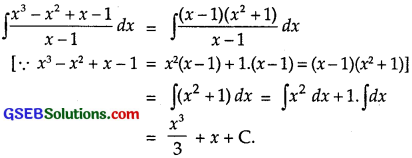Question 14.
∫(1 – x)$$\sqrt{x}$$ dx
Solution: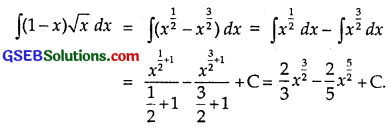Question 15.
∫$$\sqrt{x}$$(3x2 + 2x + 3)dx
Solution: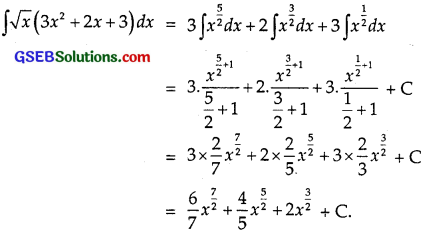Question 16.
∫(2x – 3cosx + ex)dx
Solution: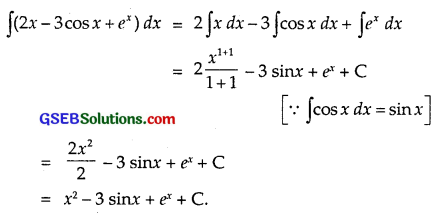Question 17.
∫(2x2 – 3sinx + 5$$\sqrt{x}$$)dx
Solution: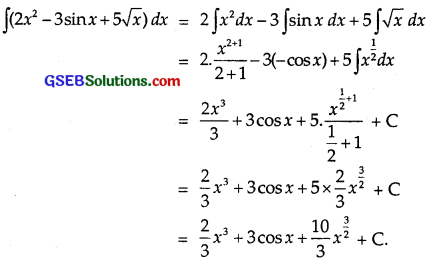Question 18.
∫secx(sec x + tan x)dx
Solution: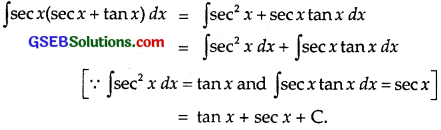Question 19.
∫$$\frac{sec^{2}x}{cosec^{2}x}$$dx.
Solution: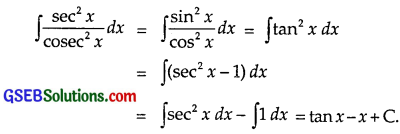Question 20.
∫$$\frac{2-3 \sin x}{\cos ^{2} x}$$ dx.
Solution: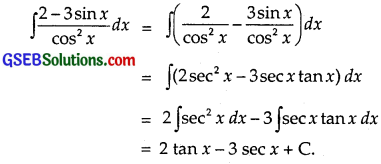Choose the correct answers in the following questions 21 and 22:
Question 21.
The antiderivative of ($$\sqrt{x}$$ + $$\frac{1}{\sqrt{x}}$$) equals
(A) $$\frac{1}{3}$$x1/3 + 2x1/2 + C
(B) $$\frac{2}{3}$$x2/3 + $$\frac{1}{2}$$x2 + C
(C) $$\frac{2}{3}$$x3/2 + 2x1/2 + C
(D) $$\frac{3}{2}$$x3/2 + $$\frac{1}{2}$$x1/2 + C
Solution: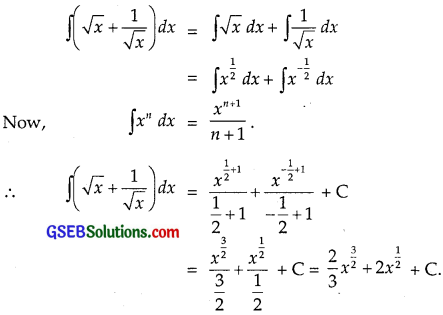⇒ Part(C) is the correct answer.

Question 22.
If $$\frac{d}{dx}$$ f(x) = 4x3 – $$\frac{3}{x^{4}}$$ such that f(2) = 0, then f(x) is
(A) x4 + $$\frac{1}{x^{3}}$$ – $$\frac{129}{8}$$
(B) x3 + $$\frac{1}{x^{4}}$$ + $$\frac{129}{8}$$
(C) x4 + $$\frac{1}{x^{3}}$$ + $$\frac{129}{8}$$
(D) x3 + $$\frac{1}{x^{4}}$$ – $$\frac{129}{8}$$
Solution: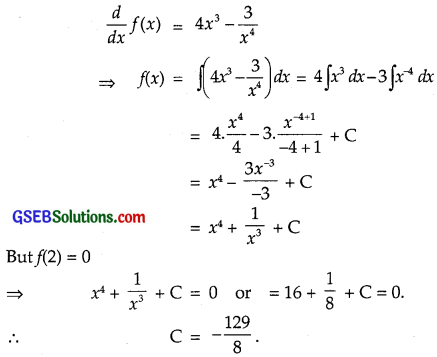Putting C = – $$\frac{129}{8}$$ in (1), we get
f(x) = x4 + $$\frac{1}{x^{3}}$$ – $$\frac{129}{8}$$
⇒ Part(A) is the correct answer.